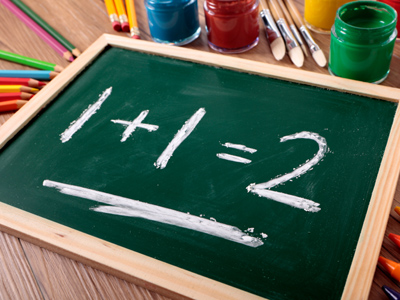One plus one equals two.

# Which Symbol Should We Use? - Addition, Subtraction and Equals Signs

This Math quiz is called 'Which Symbol Should We Use? - Addition, Subtraction and Equals Signs' and it has been written by teachers to help you if you are studying the subject at elementary school. Playing educational quizzes is a fun way to learn if you are in the 1st or 2nd grade - aged 6 to 8.

It costs only \$12.50 per month to play this quiz and over 3,500 others that help you with your school work. You can subscribe on the page at Join Us

Recognising, selecting and using the right symbol in a number sentence is vital. A good understanding of what the addition, subtraction and equals signs look like, what they mean in math, and what they do to numbers is a really important area of development. Understanding the language associated with the addition, subtraction and equals signs will help children calculate accurately.

How much do you know about the symbols used in math?

1.
Which symbol could be used instead of the words in brackets?
5 + 4 (is equal to) 9
+
=
-
x
This symbol means 'is equal to' or 'is the same as'
2.
Which symbol is missing from this calculation:
4 ? 1 = 3
÷
=
+
-
Subtracting 1 from 4 gives an answer of 3
3.
What does this symbol mean: -
Multiplication
Subtracting or subtraction
Division
This symbol is used in subtraction calculations, such as 5 - 3 = 2
4.
Which symbol is missing from this calculation:
3 ? 6 = 9
+
-
=
x
5.
What is wrong with this number sentence:
7 + 3 = 4
The addition sign should be a subtraction sign
The addition sign is in the wrong place
The equal sign is in the wrong place
The numbers are too small
If you change - for +, the answer becomes correct
6.
Which symbol is missing from this calculation:
6 - 2 ? 4
x
+
=
-
6 - 2 is equal to, or the same as 4
7.
Which symbol could be used instead of the words in brackets?
5 (minus) 5 = 0
x
=
+
-
Minus is another way of saying subtract or take away
8.
What does this symbol mean: =
Is equal to or the same as
Dividing
Multiplying
Many people think this symbol means 'is the answer' but it really means 'is equal to'
9.
Which symbol could be used instead of the words in brackets?
5 (plus) 5 = 10
-
=
+
÷
Plus is another word for add
10.
What does this symbol mean: +